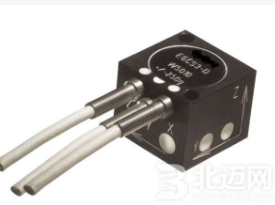# Analysis of the working principle of linear accelerometer

An acceleration sensor is an electronic device that can measure acceleration forces. Acceleration force is the force that acts on an object during acceleration, just like the gravity of the earth, which is gravity. The acceleration force can be a constant, such as g, or a variable. There are two types of accelerometers: one is an angular accelerometer, which is an improvement of a gyroscope (angular velocity sensor). The other is a linear accelerometer.

By measuring acceleration due to gravity, you can calculate the tilt angle of the device relative to the horizontal plane. By analyzing dynamic acceleration, you can analyze how the device moves. But at the beginning, you may find that measuring light tilt and acceleration is not very useful. But now engineers have come up with many ways to get more useful information.Acceleration sensors can help your robot understand the environment it is in. Are you climbing? Still walking downhill and falling? Or for flying robots, it is also crucial to control attitude. What's more important is that your robot didn't take the bomb to the crowded place by itself. A good programmer can use acceleration sensors to answer all of the above questions. Acceleration sensors can even be used to analyze engine vibration.

The principle of a linear accelerometer is the principle of inertia, which is the balance of forces. A (acceleration) = F (inertial force) / M (mass) We only need to measure F. How to measure F? Just use electromagnetic force to balance this force. Then we can get the relationship between F and current. It is only necessary to use experiments to calibrate this scale factor. Of course, the intermediate signal transmission, amplification, and filtering are the matter of the circuit.

Most accelerometers work according to the principle of piezoelectric effect. The so-called piezoelectric effect is "external force on a crystal of a heteropolar crystal that does not have a center of symmetry. In addition to deforming the crystal, it will also change the polarization state of the crystal. An electric field is established inside the crystal. This phenomenon of polarizing the medium due to mechanical force is called the positive piezoelectric effect. "

Generally, an acceleration sensor uses the characteristic of crystal deformation caused by acceleration in its interior. Because this deformation generates a voltage, as long as the relationship between the generated voltage and the applied acceleration is calculated, the acceleration can be converted into a voltage output. Of course, there are many other ways to make acceleration sensors, such as piezoresistive technology, capacitive effect, thermal bubble effect, light effect, but the most basic principle is that a certain medium is deformed due to acceleration. By measuring the amount of deformation and using The relevant circuit is converted into a voltage output. Each technology has its own opportunities and problems.

Piezoresistive acceleration sensors have developed the fastest due to their wide application in the automotive industry. Acceleration sensors are mainly used in safety performances such as automotive airbags, anti-lock braking systems, and traction control systems. As safety is increasingly becoming a selling point for automakers, such additional systems are also increasing. The market size of piezoresistive acceleration sensors in 2000 was about US \$ 420 million. According to relevant surveys, its market value is expected to grow at an average annual rate of 4.1%, reaching US \$ 560 million in 2007. Among them, the European market is the fastest, because Europe is home to many airbag and car manufacturers.